# Thread: Differentiating a function

1. ## Differentiating a function

Hey Guys, I'm working on the Properties of Derivatives and am having trouble with 2 of my homework questions:

Differentiate the following functions:
d) f(x) = sqrt (x) - ^3 sqrt (x)

and

e) f(x) = 3x / x^2 + 4

Sorry I don't know how to use LaTeX, if you can't understand the questions I took a picture of them as well.
http://i616.photobucket.com/albums/t...s/102_6285.jpg

Thank you so much for any help I can get!

OH also, when I'm calculating the derivative using the first principle definition of f(x) = sqrt (2x)
How do I start the process? Is it f(x) = sqrt (2x+h) or sqrt 2(x+h)

2.Originally Posted by lil_cookieHey Guys, I'm working on the Properties of Derivatives and am having trouble with 2 of my homework questions:

Differentiate the following functions:
d) f(x) = sqrt (x) - ^3 sqrt (x)

and

e) f(x) = 3x / x^2 + 4

Sorry I don't know how to use LaTeX, if you can't understand the questions I took a picture of them as well.
http://i616.photobucket.com/albums/t...s/102_6285.jpg

Thank you so much for any help I can get!

OH also, when I'm calculating the derivative using the first principle definition of f(x) = sqrt (2x)
How do I start the process? Is it f(x) = sqrt (2x+h) or sqrt 2(x+h)
(d) $\displaystyle f(x) = \sqrt{x} - \sqrt{x} = x^{1/2} - x^{1/3}$. Differentiate in the usual way.

(e) $\displaystyle f(x) = \frac{3x}{x^2 + 4}$. Differentiate using the quotient rule.

$\displaystyle f(x) = \sqrt{2x} \Rightarrow f(x+h) = \sqrt{2(x+h)}$.

3. I got the first question and the last one but I'm still confused about question (e)

could someone please give me a little more help with this.....thanks

when I attempted to answer it I got:

3x^3 - 6x^2
-------------
(x^2 + 4)^2

4. Just in case a picture helps...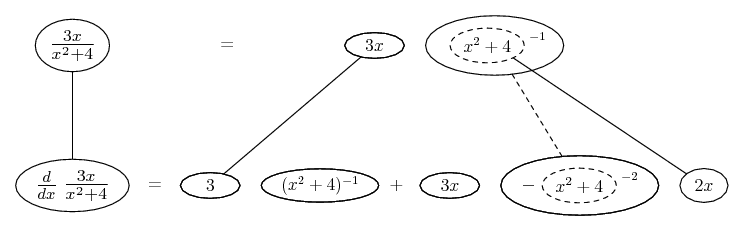Straight lines differentiate downwards with respect to x, the straight dashed line with respect to the dashed balloon expression, for the sake of the chain rule. And the chain rule (for the power of minus one) operates inside of the product rule. The quotient rule comes out of this, but sometimes it helps to see it this way. Hope so - but someone will no doubt post something more orthodox, so fear not!

Balloon Calculus: worked examples from past papers

5. By the way the quotient rule is a bit more evident here if we tweak a couple of the expressions...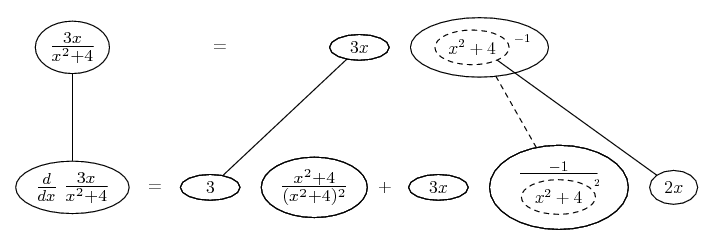And you may want isolate the triangular network that shows the chain rule...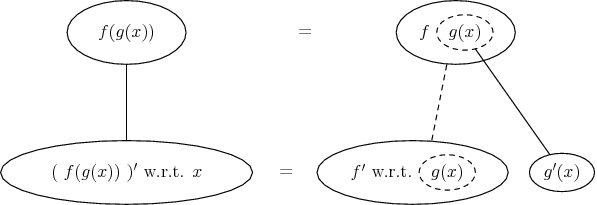And the network that shows the product rule...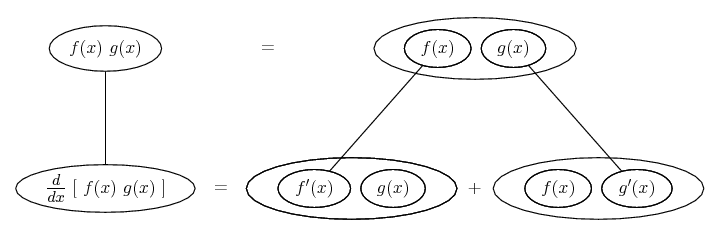... which we can highlight in the big picture, if we want to (a matter of taste) by enclosing some of the balloons in some more.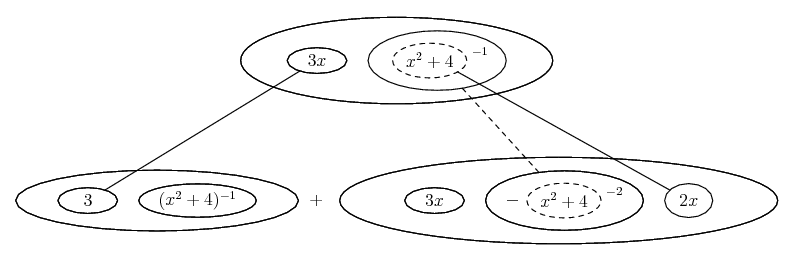Don't integrate - balloontegrate!

Balloon Calculus: worked examples from past papers

#### Search Tags

differentiating, function